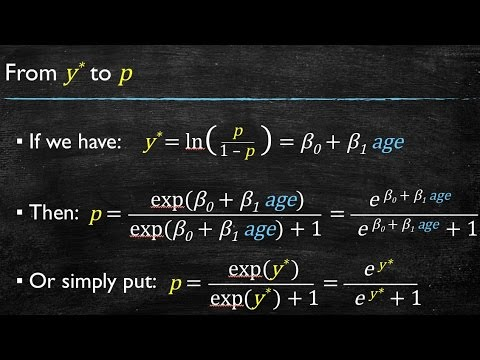1629180621

## Logistic Regression Details Pt 2: Maximum Likelihood

This video follows from where we left off in Part 1 in this series on the details of Logistic Regression. This time we’re going to talk about how the squiggly line is optimized to best fit the data.

NOTE: In statistics, machine learning and most programming languages, the default base for the log() function is 'e'. In other words, when I write, "log()", I mean "natural log()", or "ln()". Thus, the log to the base 'e' of 2.717 = 1.

#statquest #logistic #MLE #machine-learning1626662647

## Logistic Regression - Interpretation of Coefficients and Forecasting

This video discusses the interpretation of a logistic regression’s coefficients and, more specifically, the slope of the independent variables when all other variables are held at their means. We also show evidence of the non-linear relationship between the independent variables and the dependent variable.

00:00 Introduction
00:21 Recap of Logistic Regression
01:10 Leveraging the Similarities with Linear Models
02:15 What changed?
03:06 From y* to p
04:03 From y* to p in Excel (Simple Regression)
10:18 Logistic Model Plot
10:54 Multiple Logistic Regression
11:30 Result of Multiple Logistic Regression
12:36 From y* to p in Excel (Multiple Regression)

👕 T-shirts for programmers: https://bit.ly/3ir3Gci

#logistic #python1626511199

## Logistic Regression Details: Coefficients - Part 1

When you do logistic regression you have to make sense of the coefficients. These are based on the log(odds) and log(odds ratio), but, to be honest, the easiest way to make sense of these are through examples. In this StatQuest, I walk you though two Logistic Regression Examples, step-by-step, and show you exactly how the coefficients are derived and how to interpret them.

NOTE: In statistics, machine learning and most programming languages, the default base for the log() function is ‘e’. In other words, when I write, “log()”, I mean “natural log()”, or “ln()”. Thus, the log to the base ‘e’ of 2.717 = 1.
ALSO NOTE: Starting at 15:21, the left hand side of the equation should be “log(odds Obesity)” instead of “size”.

0:00 Awesome song and introduction
1:13 Review of Logistic Regression Concepts
2:47 Coefficients for continuous variables
10:46 Coefficients for discrete variables
17:52 Coefficients for combinations of variable types

#logistic #machinelearning1592427660

## Data Science Live - 3 | Logistic Regression in Python | Python Training

Video on Logistic Regression in Python will give you basic understanding of Logistic Regression Machine Learning Algorithm with examples. In this video, you will also get to see demo on Logistic Regression using Python.

#data science #logistic #python